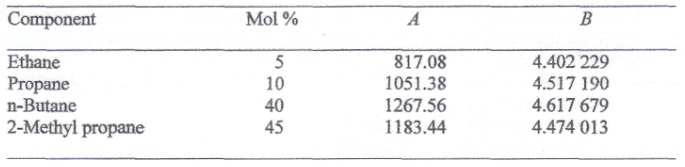Molarity

what is the molarity of the solution prepared by dissolving 75.5 g of pure KOH in 540 ml of solution

#### Related Questions in Chemistry

• ##### Q :Relative reactivity Which is more

Which is more reactive towards nucleophilic substitution aryl halide or vinyl halides

• ##### Q :Molal elevation constant of water The

The boiling point of 0.1 molal aqueous solution of urea is 100.18oC  at 1 atm. The molal elevation constant of water is: (a) 1.8    (b) 0.18   (c) 18    (d) 18.6

• ##### Q :Application of colligative properties

Choose the right answer from following. Colligative properties are used for the determination of: (a) Molar Mass (b) Equivalent weight (c) Arrangement of molecules (d) Melting point and boiling point (d) Both (a) and (b)

• ##### Q :Problem on partial pressure i) Show

i) Show that the equilibrium constant Kp for the reaction

CaCo3(s) ↔ CaO(s) +CO2(g)

is about unity (i.e. = 1.0) at 895 °C.

ii) If two grams of calcium carbonate are pl

• ##### Q :Effect of addition of mercuric iodide

Give me answer of this question. When mercuric iodide is added to the aqueous solution of potassium iodide, the:
(a) Freezing point is raised (b) Freezing point is lowered (c) Freezing point does not change (d) Boiling point does not change

• ##### Q :Problem on bubble point The following

The following mixture of hydrocarbons is obtained as one stream in a petroleum refinery.##### Q :Colligative property associated question

Give me answer of this question. Which of the following is not a colligative property : (a)Optical activity (b)Elevation in boiling point (c)Osmotic pressure (d)Lowering of vapour pressure

• ##### Q :Avogadro's hypothesis Law Principle

Avogadro's hypothesis Law Principle- Berzelius, a chemist tried

• ##### Q :Describe Transformation Matrices. Each

Each symmetry operation can be represented by a transformation matrix.

You have seen what happens when a molecule is subjected to the symmetry operation that corresponds to any of the symmetry elements of the point group to which the molecule belongs. The m

• ##### Q :Problem associated to vapour pressure

Provide solution of this question. 60 gm of Urea (Mol. wt 60) was dissolved in 9.9 moles, of water. If the vapour pressure of pure water is P0 , the vapour pressure of solution is:(a) 0.10P0 (b) 1.10P0 (c) 0.90P0 (d) 0.99P0